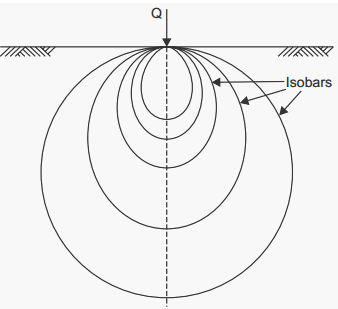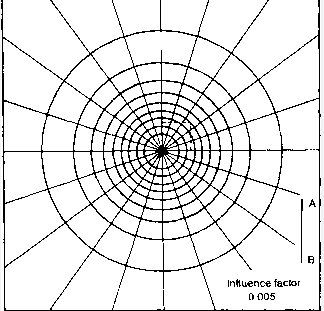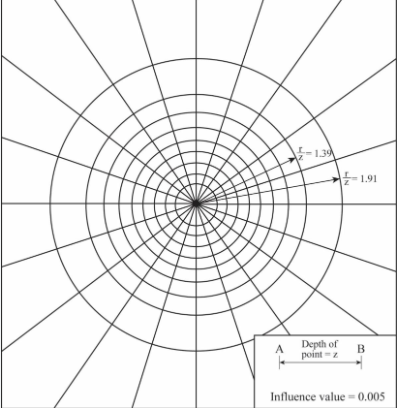Select Page

# Stresses due to Applied Load

Contents

Recall the Vertical Stresses under various types of load such as: i. Point Load ii. Line Load iii. Uniformly Loaded Circular Area iv. Uniformly Loaded Rectangular Area 1

Recall Isobar Diagram and the Influence Diagram 2

Recall the Newmark’s Influence Chart 4

Describe the Westergaard’s Equation 5

Recall the Contact Pressure Distribution 6

# Recall the Vertical Stresses under various types of load such as: i. Point Load ii. Line Load iii. Uniformly Loaded Circular Area iv. Uniformly Loaded Rectangular Area

1. Point Load: A point load is a concentrated load applied at a single point on a soil or rock mass. The vertical stress produced by a point load is given by the equation, σ = q/A, where q is the magnitude of the point load, and A is the area over which the load is applied. The vertical stress produced by a point load is directly proportional to the magnitude of the load and inversely proportional to the area over which the load is applied.
4. Uniformly Loaded Rectangular Area: A uniformly loaded rectangular area is an area on a soil or rock mass that is loaded with a uniform load over its entire surface. The vertical stress produced by a uniformly loaded rectangular area is given by the equation, σ = q/A, where q is the magnitude of the uniform load, and A is the area of the rectangular area over which the load is applied. The vertical stress produced by a uniformly loaded rectangular area is directly proportional to the magnitude of the load and inversely proportional to the area of the rectangular area over which the load is applied.

It is important to note that the above equations are valid for isotropic and homogeneous soils and rock masses and that the actual stresses on the ground may vary depending on the soil and rock type, condition, and the location of the load.

# Recall Isobar Diagram and the Influence Diagram

1. Isobar Diagram: An isobar diagram is a graphical representation that is used to depict the variation of vertical stress with depth in a soil or rock mass. The isobar diagram is used to study the distribution of vertical stress in a soil or rock mass and the influence of different loads on the soil or rock mass. The isobar diagram is constructed by plotting the vertical stress at various depths in the soil or rock mass, and the lines of equal vertical stress are called isobars. The isobar diagram is useful in understanding the distribution of stress in a soil or rock mass and can be used to determine the factor of safety of a slope, foundation or other structure.1. Influence Diagram: An influence diagram is a graphical representation that is used to depict the variation of vertical stress with distance from a load. The influence diagram is used to study the distribution of vertical stress in a soil or rock mass due to a specific load. The influence diagram is constructed by plotting the vertical stress at various distances from the load, and the lines of equal vertical stress are called isobars. The influence diagram is useful in understanding the distribution of stress in a soil or rock mass due to a specific load and can be used to determine the factor of safety of a slope, foundation or other structure.The Isobar and Influence diagrams are very useful for geotechnical engineers to understand the distribution of stress in soil and rock masses. They are commonly used in the design of foundations, slopes, and embankments and can be used to determine the factor of safety of these structures. It is important to note that these diagrams are based on the assumptions of isotropic and homogeneous soils and rock masses and that the actual stresses on the ground may vary depending on the soil and rock type, condition, and the location of the load.

# Recall the Newmark’s Influence Chart

The Newmark’s Influence Chart is a graphical representation that is used to determine the distribution of vertical stress in a soil or rock mass due to a specific load. It is also known as “Newmark’s Slip Circle Method”. It is based on the assumption that the soil or rock mass is isotropic and homogeneous. The chart is divided into four quadrants, and each quadrant represents a different type of load: point load, line load, circular load, and rectangular load.

1. The first quadrant represents a point load. The chart shows the distribution of stress around the point load in terms of radial stress and tangential stress. The radial stress is the stress perpendicular to the load, while the tangential stress is the stress parallel to the load.
2. The second quadrant represents a line load. The chart shows the distribution of stress along the line load in terms of vertical stress and horizontal stress. The vertical stress is the stress perpendicular to the line load, while the horizontal stress is the stress parallel to the line load.
3. The third quadrant represents a circular load. The chart shows the distribution of stress around the circular load in terms of radial stress and tangential stress. The radial stress is the stress perpendicular to the load, while the tangential stress is the stress parallel to the load.
4. The fourth quadrant represents a rectangular load. The chart shows the distribution of stress within the rectangular load in terms of vertical stress and horizontal stress. The vertical stress is the stress perpendicular to the rectangular load, while the horizontal stress is the stress parallel to the rectangular load.The Newmark’s Influence Chart is widely used in geotechnical engineering to determine the distribution of stress in soil and rock masses. It is commonly used in the design of foundations, slopes, and embankments and can be used to determine the factor of safety of these structures. It is important to note that the chart is based on the assumptions of isotropic and homogeneous soils and rock masses and that the actual stresses on the ground may vary depending on the soil and rock type, condition, and the location of the load.

# Describe the Westergaard’s Equation

Westergaard’s equation is a mathematical equation that is used to determine the radial stress and tangential stress in a soil or rock mass due to a point load. The equation is based on the assumption that the soil or rock mass is isotropic and homogeneous. The equation can be used to determine the distribution of stress around a point load, such as a foundation or a load bearing wall.

The equation is given by:

σ r = (P / (2πr)) * (1 + 2r/R)

σ t = (P / (2πr)) * (1 – r/R)

Where:

σ = tangential stress

r = distance from the point load to the point of interest

The Westergaard’s equation is widely used in geotechnical engineering to determine the distribution of stress in soil and rock masses. It is commonly used in the design of foundations, slopes, and embankments and can be used to determine the factor of safety of these structures. It is important to note that the equation is based on the assumptions of isotropic and homogeneous soils and rock masses and that the actual stresses on the ground may vary depending on the soil and rock type, condition, and the location of the load.

This equation is useful in understanding the distribution of stresses in soil and rock masses. It is also used for the design of foundations, slope stability analysis and embankments etc. It is important to note that the equation is based on the assumptions of isotropic and homogeneous soils and rock masses and that the actual stresses on the ground may vary depending on the soil and rock type, condition, and the location of the load.

# Recall the Contact Pressure Distribution

Contact pressure distribution refers to the distribution of pressure that is exerted on the soil or rock mass by a foundation or load-bearing structure. This pressure is the result of the weight of the structure and any additional loads that are applied to it. The contact pressure distribution is an important factor in the design of foundations and other load-bearing structures, as it affects the stability and settlement of the structure.

There are several ways to calculate contact pressure distribution, including the following:

1. Strip foundation: Strip foundations are used when the loads are applied along a line, such as a wall. The contact pressure is assumed to be linear, with the highest pressure at the center of the foundation and the lowest pressure at the edges.
2. Pad foundation: Pad foundations are used when the loads are applied over an area, such as a column. The contact pressure is assumed to be circular, with the highest pressure at the center of the foundation and the lowest pressure at the edges.
3. Floating foundation: A floating foundation is a type of foundation that is used when the soil or rock mass is not capable of supporting the loads. The contact pressure is zero, as the foundation is not in contact with the soil or rock mass.
4. Plate load test: The plate load test is a field test that is used to determine the contact pressure distribution. A steel plate is placed on the soil or rock mass, and a load is applied to the plate. The load is then increased until failure, and the contact pressure distribution is determined from the load and deflection data.

The contact pressure distribution is affected by several factors, including the type of soil or rock mass, the shape and size of the foundation, and the loads that are applied to the structure. It is important to consider the contact pressure distribution when designing foundations and other load-bearing structures, as it affects the stability and settlement of the structure.

It is important to note that the actual stresses on the ground may vary depending on the soil and rock type, condition, and the location of the load. The contact pressure distribution is calculated based on the assumptions of isotropic and homogeneous soils and rock masses, however, the actual distribution of stresses may vary depending on the soil and rock type, condition, and the location of the load.